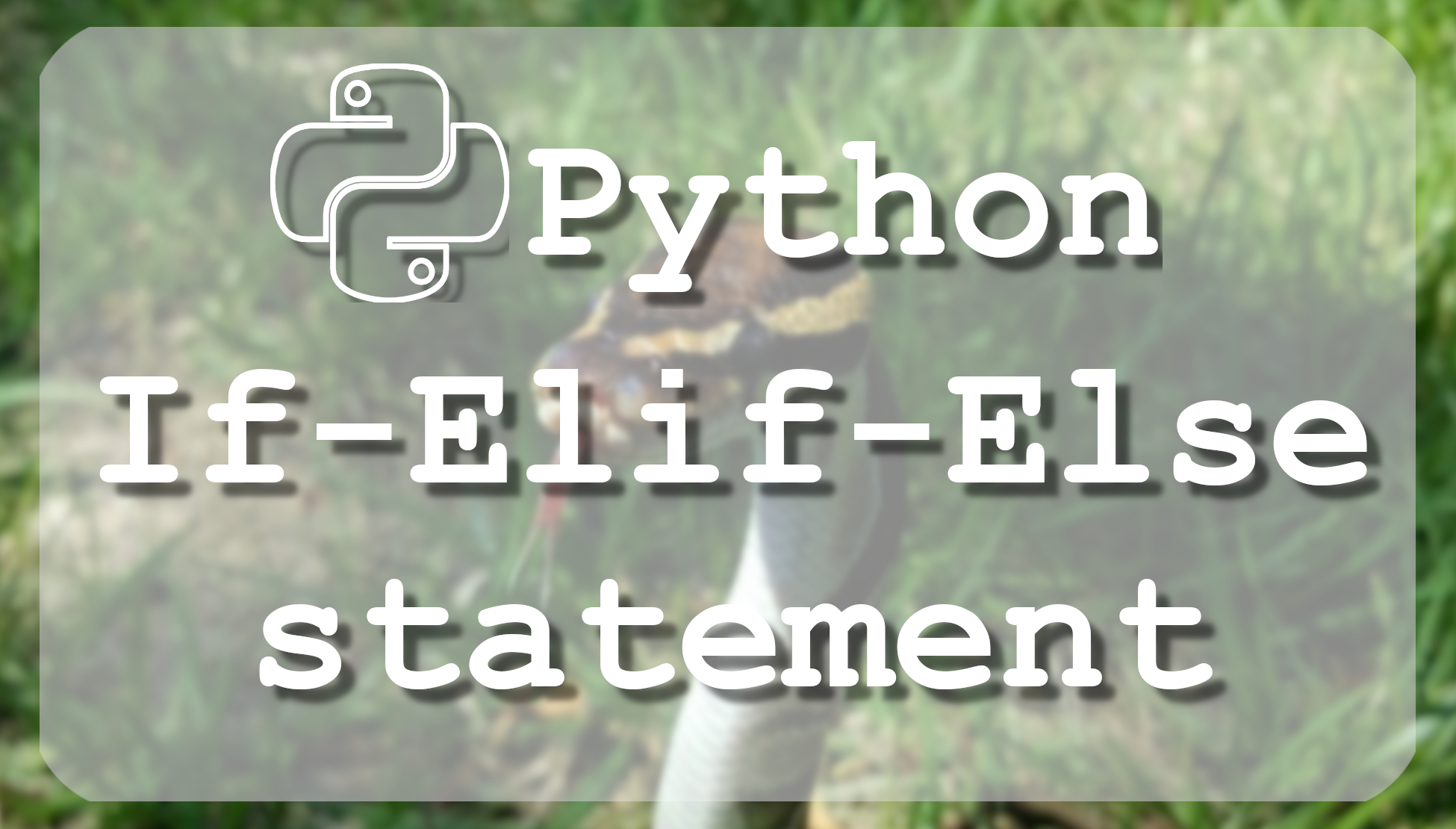# Tutorial - Python 3 basics If Elif Else statements

## This tutorial is about Python for loop, short basic introduction with for loop syntax and how to use range loop.

If you are already familiar with programming, probably you already heard about the "If" statement which is one of the most basic forms of logic that can be introduced. The idea of the if statement is to assess whether something is the case, and, if it is, then to perform the following block of code within the statement.
The if statement contains a logical expression using which data is compared and a decision is made based on the result of the comparison.

## IF statement syntax:

``````if expression:
statement(s)``````

If the expression evaluates to TRUE, then the block of the statement(s) inside the if statement is executed. If an expression evaluates to FALSE, then the first set of code after the end of the if statement(s) is executed.

If statement example:

``````a = 10
b = 20

if a < b:
print("a is less than b")``````

Here, we defined a and b. Then, we asked if b is greater than a. If it is, then we will print "a is less than b".

## Python If-Else Statement:

An else statement can be combined with an if statement to build the logic. An else statement contains the block of code that executes if the conditional expression in the if statement resolves to a FALSE value.
The else statement is an optional statement and there could be at most only one else statement following if.

Syntax:

``````if expression:
statement(s)
else:
statement(s)``````

If-Else statement example:

``````a = 10
b = 20

if a > b:
print("a is more than b")
else:
print("a is less than b")``````

When the above code is executed, we ask if a is greater than b. If it's not, then else statement runs.

## If-Elif-Else Statement:

The `elif `statement allows checking multiple expressions for TRUE and executing a block of code as soon as one of the conditions evaluates to TRUE. Similar to the else, the `elif` statement is optional. However, unlike else, for which there can be at most one statement, there can be an arbitrary number of `elif` statements following one if.

###### Syntax:
``````if expression1:
statement(s)
elif expression2:
statement(s)
...
else:
statement(s)``````

Core Python does not provide a switch or case statement as in other languages, but we can use if, elif, or else statements to simulate switch case by inserting elif statements as much as we want.

If-Elif-Else statement example:

``````a = 10
b = 20
c = 0

if a > b:
print("a is more than b")
elif a < c:
print("a is less than c")
else:
print("there was something wrong")``````

Here, we asked if a is greater than b first. 10 is not greater than 20, so this is False. So the `elif` runs to ask if a is less than c. In this case, it is asking if 0 is less than 10. It is not, so we find ourselves at the else statement which notifies us that "there was something wrong".

## Nested If statement:

There may be a situation when you want to check for another condition after a condition resolves to true. In such a situation, you can use the nested if construct.

Syntax:

``````if expression1:
statement(s)
if expression2:
statement(s)
elif expression3:
statement(s)
else:
statement(s)
elif expression4:
statement(s)
else:
statement(s)``````

In a nested, if construct, you can have an if, elif, else construct inside another if, elif, else construct.

Nested statement example:

``````a = 10
b = 20
c = 0

if a > b:
print("a is more than b")
elif b > c:
if a == b:
print("a is equal to b")
else:
print("a isn't equal to b")
else:
print("there was something wrong")``````

Here is just a basic example where we defined a, b and c. Then, we asked if a is greater than b. If it is, then we will print "a is more than b", but it's not. Then we go to `elif` statement, where we check if b is greater than c. It is greater, so then we enter a new if statement, where we check if a is equal to b, but it's not. So in this case we move to `else` statement, where we say that a is not equal to b.

In this tutorial, we covered `if, elif, else` and nested statements, now you can start creating your own logic in statements